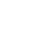2020年成人高考高起点《数学》知识点大集合

交集和并集

1、取集合A和集合B的公共部分，记作A∩B。

2、取集合A和集合B的全部元素，记作A∪B。

简单逻辑

1、充分条件：如果A成立，那么B成立，“A推出B，B不能推出A”。

2、必要条件：如果B成立，那么A成立，“B推出A，A不能推出B”。

3、充要条件：如果A→B，又有A←B，“A推出B，B推出A”。

函数部分

1、绝对值的不等式

绝对值不等式的解法:

|ax+b|

(当a<0的时候，不等号要改变方向

|ax+b|>c相当于解不等式ax+b>c或ax+b<-c

2、常见函数的定义域

3、函数的单调性

第一种方法用取值法:任取2个数x1.x2.且x1

若f(x1)f(x2)，则为减函数。

第二种方法用求导法(见后面)。

4、函数的奇偶性

令x=-x，若f(-x)=-f(x)，则f(x)为奇函数;

若f(-x)=f(x)，则f(x)为偶函数。

向量和直线

1、向量

设a=(x1.y1)b=(x2.y2)，则:

加法运算:a+b=(x1.y1)+(x2.y2)=(x1+x2.y1+y2)

减法运算:a-b=(x1.y1)-(x2y2)=(x1-x2:y1-y2)

数乘运算:ka=k(x1.y1)=(kx1.ky1)

内积运算:a*b=(x1.y1)(x2.y2)= x1x2 +y1y2

垂直向量:a⊥b= x1x2 +y1y2=0

平行向量:a//b= x1y2 +x2y1=0

2、直线方程的几种形式(记住其中一种就可以)

点斜式:y-yo=k(x-x0)，已知斜率k和某点坐标(xo,yo)

斜截式:y=kx+b，已知斜率k和在y轴的截距b

绝对值不等式的解法:

|ax+b|

(当a<0的时候，不等号要改变方向)

|ax+b|>c,相当于解不等式ax+b>c或ax+b<-c

导数的应用

1、导数的几何意义

(1)几何意义:函数f(x)在点(x0.y0)处的导数值f'(x0)，即为f(x)在点(x0.y0)处切线的斜率。

(2)常用导数公式:c为常数

2、函数单调性

f'(x)>0则f(x)在(a，b)内严格单调增加

f'(x)<0则f(x)在(a，b)内严格单调减少。

3、函数的极值、最大值、最小值

f'(x)=0的点----函数f(x)的驻点。设为x0

(1)若x< x0时，f'(x)>0;x> x0时，f'(x)<0.则f(x0)为f(x)的极大值点。

(2)若x

(3)如果f'(x)在x0的两侧的符号相同，那么f(x0)不是极值点。

(4)极值和端点的函数值中最大和最小的就是最大值和最小值。### 在线测试

• 模拟试题
• 历年真题#### 免费在线咨询#### 微信咨询

18961806279#### 免费咨询热线

400-1886-017### 首页